# Weibull examples - HxGN EAM - 11.07.01 - Feature Briefs - Hexagon

## HxGN EAM Reliability Analysis

Language
English
Product
HxGN EAM
Search by Category
Feature Briefs
HxGN EAM Version
11.7.1

In this first example there are a total of 6 observations with a time to failure of 34, 16, 120, 53, 75 and 93. For this population β = 1.42696711 and η = 76.3454156.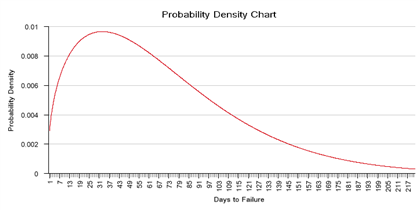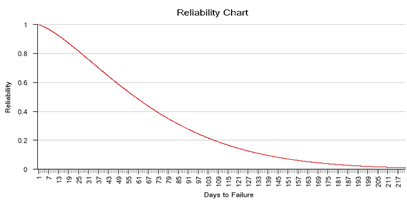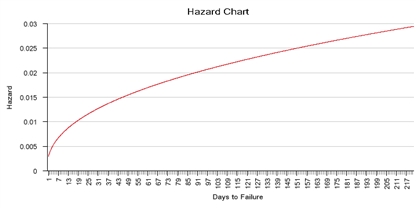One of the characteristics of the Weibull distribution is that η represents the age at which 63.2 percent of the population has failed. You can see this represented on the Reliability function. Note that reliability is the complement of F(x) so 76 days intercepts at 36.8 percent. At 40 days 60 percent is still alive, at 200 days all have pretty much failed.

The hazard function clearly indicates that as age increases the chance for failure also increases, indicating wear and tear. You will always find this correlation if β > 1.

To determine if the data "matches" the distribution and the calculated parameters you can plot the probability. The general idea is to take the model Cumulative distribution function equation and write it in such a way that a function of F(x) is a linear equation of a function of x. For the Weibull CDF this means the y-axis will be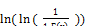and the x-axis will be ln(x). After plotting this graph and if the data is consistent with the Weibull distribution, then the points will line up roughly on a straight line with slope = β. For the x-axis the F(x) can be approximated using the median rank equation of Benard’s,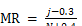where j is the failure order or sequence and N is the total number of failures.

In another example there are a total of 6 observations with a time to failure of 15, 240, 10, 120, 35, and 25. For this population β = 0.8139495 and η = 73.0026692. On the Reliability Calculations tab of the equipment screens, you can now view the following reports: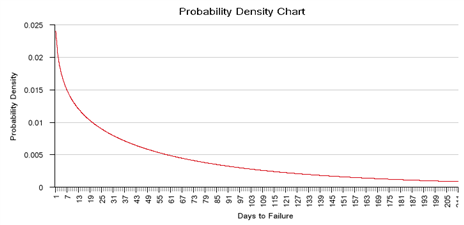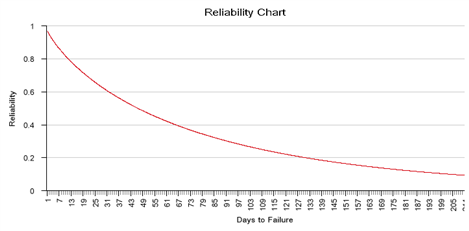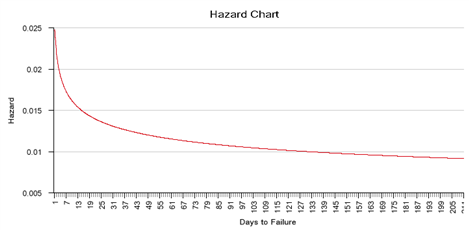The hazard function clearly indicates that as age increases the chance for failure decreases, indicating infant mortality. You will always find this correlation if β < 1. The probability function also clearly indicates that the chance for failure decreases with age.

When β = 3.5 the Weibull distribution is a perfect normal distribution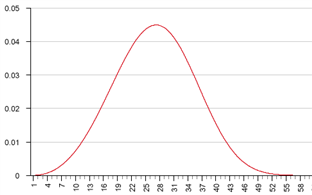When β = 1 the failures are random, and the hazard function therefore is flat.